# TensorFlow 2. Shadow CNN example for MNIST data

The practice is to understand how Tensorflow applied to shadow NN in MNIST data. The practice is from Big Data University lectures.

1. Reference:

Support_Vector_Machines.html  (Coursera Machine Learning Course)

Big Data University TensorFlow course

1. Deep Learning Concept
1.  Using multiple process layer with non-linear algorithm to simulate brain ability;
2.  A branch of machine learning.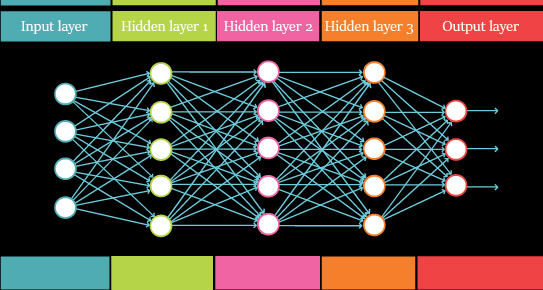We will focus on shadow NN in this note.

1. Shadow NN MNIST Example: two or three layers only.

In the context of supervised learning, digits recognition in our case, the learning consists of a target/feature which is to be predicted using a given set of observations with the already known final prediction (label).

1. the target will be the digit (0,1,2,3,4,5,6,7,8,9)
2. the observations are the intensity and relative position of pixels
3. After some training, it’s possible to generate a “function” that map inputs (digit image) to desired outputs (type of digit).

1. Our data: MNIST

MNIST is a: “database of handwritten digits that has a training set of 60,000 examples, and a test set of 10,000 examples. It is a subset of a larger set available from NIST.

*1)The digits have been size-normalized and centered in a fixed-size image”

*2) MNIST is a high optimized data-set and it does not contain images. build your own code if you want to see the real digits.

1. Data presentation of digital label: 0, 1,2,3,…9

1. Binary:  such as 3 is represented as 0011, 4 is 0100,9 is 1001
2. One-hot:  one bit will be on for a specific digit.

Number representation:    0

One-hot encoding:                          

Array/vector:             0     0      0      0   0     1

Number representation:    5

One-hot encoding:                           

Array/vector:             1     0      0      0      0      0

Why one-hot?  Labelling sequence does not represent the math sequence relationship. for example, if we want to predict Apple, Pear, Bananner,Orange. In computer, all things must be number. we can make them as 1, 2, 3, 4. However, it is sequence, looks like they have math relationship. However, they are not.

So, another way is to set as “0001” as Apple, “0010” as Pear, “0100” as Banner, and 1000 as Orange. So, this representation do not have any math relationship.

from tensorflow.examples.tutorials.mnist import input_data

after that, you will get below dataset:

*1)Training (mnist.train)

– 55,000 data points

– mnist.train.images for inputs  ( Each input has 784 pixels distributed by a 28 width x 28 height matrix )

– mnist.train.labels for outputs (one_hot representation):  each output is 1×10, Indicated the label (0,1,2,3,…9)

*2)Validation (mnist.validation)

– 5,000 data points

– mnist.validation.images for inputs

– mnist.validation.labels for outputs

*3) Test (mnist.test)

– 10,000 data points

– mnist.test.images for inputs

– mnist.test.labels for outputs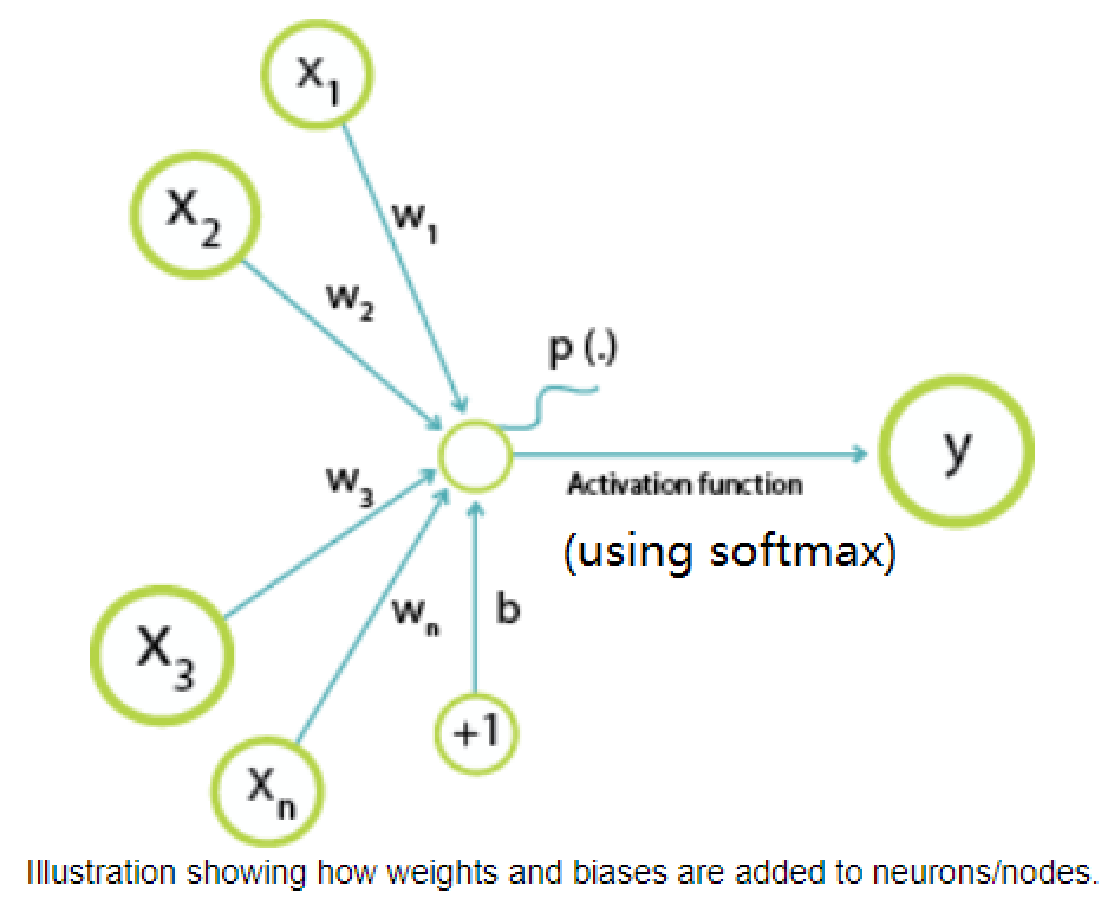1. Idea label (via human already classified, such as mnist label set) data for 9: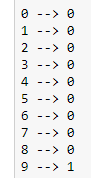1. Machine recognized with probability for 9. That can be generated by softmax function

y = tf.nn.softmax(tf.matmul(x,W) + b)

[Image_Link]https://lh3.googleusercontent.com/0EVRvJiz_Ylolwa-

1. Logistic Model

Logistic function output is used for the classification between two target classes 0/1. Softmax function is generalized type of logistic function. That is, Softmax can output a multiclass categorical probability distribution.

The core is cost function. Why cost function of logistic? Our world is constructed by ourselves. Math is to be constructed by ourselves.  How we construct our cost function of logistic?

*1) our goal:  no goal, no how.

We hope we can (of course, you can inverse below, but it makes not intuitive and more complex). So, below goal is intuitive simple, so best than inverse.

*a) make H(x) close to 1 if our predicted label is y=1; and

*b) make H(x) close to 0 if our predicted label is y=0;

*2) let’s check our sigmoid function: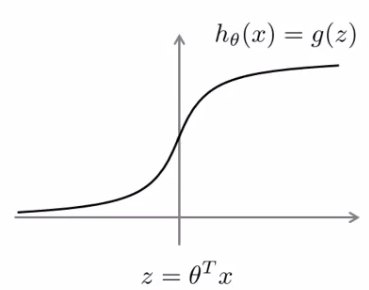So, let’s intepret our goal according to the above curve:

*a) make H(x) close to 1 if our predicted label is y=1; -> make z close to positive infinity if y=1

*b) make H(x) close to 0 if our predicted label is y=0; -> make z close to neg. infinity if y=0

so, our question is:

*c) if z is not to positive infinity when y=1, how we punish it?  (good dog, bad dog game)

*d) if z is not to neg. infinity when y=0, how we punish it?

*3) Let’s make it further for y=1 case:

Set LH(z)=-log(h(z)),  that is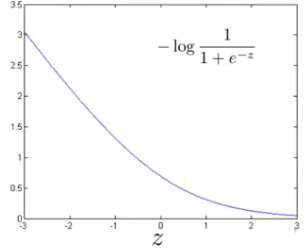Re-check our goal:  let cost=LH(z) if y=1

*a) make H(x) close to 1 if our predicted label is y=1;

-> make z close to positive infinity if y=1

->our cost close to 0 if y=1

-> that means no punishment (cost=0) if our z is close to positive infinity when y=1

-> that is intuitive make sense!!!

*4) similar for y=0 case:

Set LH(z)=-log(1-h(z)),  that is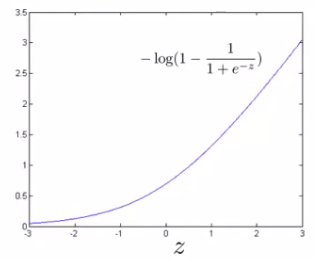Re-check our goal:  let cost=LH(z) if y=0

*b) make H(x) close to 0 if our predicted label is y=0;

-> make z close to neg. infinity if y=0

->our cost close to 0 if y=0

-> That means no punishment (cost=0) if our z is close to neg. infinity when y=0

-> That is intuitive make sense!!!

5) So, combine 3)4), we get logistics cost function: (the punishment function)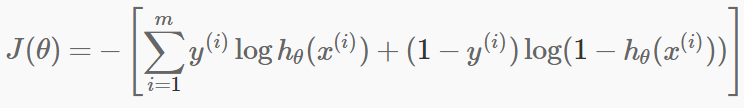1. Softmax is generation of Logistic.

Previously, H(z) (range from (0,1)) can describe how close to 0 and 1. We only need to have one set of(size is 1×784 for MNIST) to z to get H(z), then finally to punish for y=0 and y=1 accordingly. Logistic model only covers two categories.

Now, we have Softmax for K category to predict.  (K=10) for MNIST digit prediction.  We use the concept of logistic probability for Softmax model.

We can construct a parameter with dimension of Kx784, as belowFor each input x,   when multiply, we got below (the size is K, represented the normalized probability of each label.We can make it normalized probability (sum=1), and defined as function h(x)for example,if according label is 9, that is y=[0,0,0,0,0,0,0,0,0,1]

Similar as Logistic model above, we can treat h(x) asAnd we only punish for 1{y=m}=1 case (as 1{y=m}=0 cases already contribute some to 1{y=m}=1 case  via above probability normalization)

set cost to punish the probability far from 1 for the 1{y=m}=1  as below:  (for one input)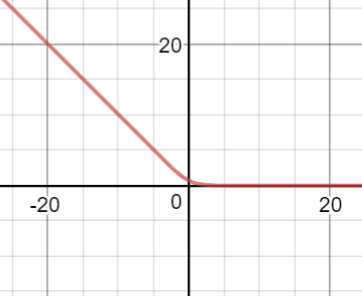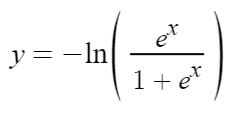So, for multiple input, we can get the Softmax formula as below:http://www.bigleaguekickball.com/about/ Cheap Soma no prescription next day delivery « (Previous News)